# Java Program to calculate simple interest

In this tutorial you will learn about the Java Program to calculate simple interest and its application with practical example.

In this tutorial, we will learn to create a Java Program to calculate simple interest  using java programming.

## Prerequisites

Before starting with this tutorial, we assume that you are best aware of the following Java programming concepts:

• Java Operators.
• Basic Input and Output function in Java.
• Class and Object in Java.
• Basic Java programming.
• If-else statements in Java.
• For loop in Java.

## What is Simple Interest?

Simple Interest is a method used in Banking , Business and in economic sectors to calculate the interest charges on loans. Formula to calculate Simple interest

### Formula:=>

1. Simple Interest = (P × R × T)/100
2. Where P = Principal Amount, R = Rate per Annum, T = Time (years)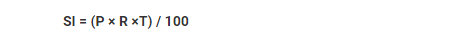where:

P is Principal amount.
R is rate per annum.
T is time in years.

### Algorithm

• Define variables as  Principal(P), Interest(i) and Time of loans in year(t).
• Use in the formula.
• Print the Simple Interest.

## Java Program to calculate simple interest

In this program we will find simple interest of given principal rate and time using  java programming. We would first declared and initialized the required variables. Next, we will find simple interest. Lets have a look at the code.

Simple Interest.

##In the above program, we have first declared and initialized a set variables required in the program.

• p= it will hold value of principal.
• r= it will hold value of interest.
• t =it will hold value of time in year.
• si= it will hold value of simple interest.

After declaring variables we initiate values in  variables.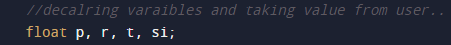now take all the values of Principal rate and time.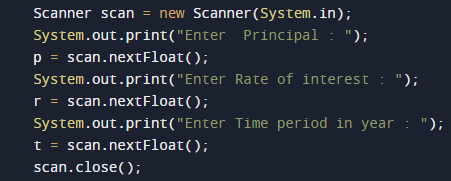after getting all the values we will calculate simple interest using the below  formula.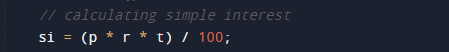Now print the simple intereset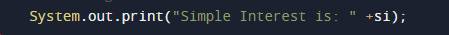In this tutorial we have learn about the Java Program to calculate simple interest and its application with practical example. I hope you will like this tutorial.